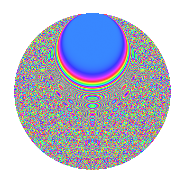# Properties

 Label 2016.2.fgLevel 2016 Weight 2 Character orbit fg Rep. character $$\chi_{2016}(19,\cdot)$$ Character field $$\Q(\zeta_{24})$$ Dimension 1264 Sturm bound 768

# Related objects

## Defining parameters

 Level: $$N$$ $$=$$ $$2016 = 2^{5} \cdot 3^{2} \cdot 7$$ Weight: $$k$$ $$=$$ $$2$$ Character orbit: $$[\chi]$$ $$=$$ 2016.fg (of order $$24$$ and degree $$8$$) Character conductor: $$\operatorname{cond}(\chi)$$ $$=$$ $$224$$ Character field: $$\Q(\zeta_{24})$$ Sturm bound: $$768$$

## Dimensions

The following table gives the dimensions of various subspaces of $$M_{2}(2016, [\chi])$$.

Total New Old
Modular forms 3136 1296 1840
Cusp forms 3008 1264 1744
Eisenstein series 128 32 96

## Trace form

 $$1264q + 4q^{2} - 4q^{4} + 12q^{5} - 8q^{7} + 16q^{8} + O(q^{10})$$ $$1264q + 4q^{2} - 4q^{4} + 12q^{5} - 8q^{7} + 16q^{8} - 12q^{10} + 4q^{11} - 24q^{14} - 8q^{16} - 12q^{19} - 8q^{22} + 12q^{23} - 4q^{25} + 12q^{26} + 12q^{28} + 16q^{29} + 4q^{32} - 16q^{35} - 4q^{37} + 12q^{38} - 12q^{40} - 32q^{43} + 44q^{44} - 4q^{46} + 24q^{47} - 8q^{50} - 84q^{52} - 12q^{53} + 48q^{56} + 28q^{58} + 108q^{59} - 12q^{61} + 56q^{64} + 8q^{65} + 4q^{67} + 12q^{68} + 28q^{70} - 48q^{71} - 12q^{73} - 12q^{74} + 8q^{77} - 8q^{79} + 48q^{80} - 132q^{82} + 24q^{85} + 4q^{86} - 48q^{88} + 12q^{89} - 56q^{91} - 48q^{92} - 84q^{94} + 152q^{98} + O(q^{100})$$

## Decomposition of $$S_{2}^{\mathrm{new}}(2016, [\chi])$$ into newform subspaces

The newforms in this space have not yet been added to the LMFDB.

## Decomposition of $$S_{2}^{\mathrm{old}}(2016, [\chi])$$ into lower level spaces

$$S_{2}^{\mathrm{old}}(2016, [\chi]) \cong$$ $$S_{2}^{\mathrm{new}}(224, [\chi])$$$$^{\oplus 3}$$$$\oplus$$$$S_{2}^{\mathrm{new}}(672, [\chi])$$$$^{\oplus 2}$$

## Hecke characteristic polynomials

There are no characteristic polynomials of Hecke operators in the database